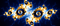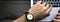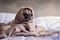# What’s So Scary About Recursion?The B in Benoit B. Mandelbrot stands for Benoit B. Mandelbrot

The Fibonacci sequence is one of the better known sequences of numbers, and one of my favourites. If you’ve never seen it — or, more likely, if you only ever saw it in some long-forgotten maths lesson and need a refresher — here are its first 10 terms:

0, 1, 1, 2, 3, 5, 8, 13, 21, 34

The Fibonacci sequence is defined by the following, recursive, function:

f(n) = f(n-1)+f(n-2)

Starting from:

f(0) = 0 & f(1) = 1

That is, after the first two terms, each successive term is the sum of the two terms before it.

If it’s clearly a recursive function, why would you use iteration to find the nth term of the Fibonacci sequence? Why reach for your trusty for loop?

Is it because recursion is hard? Let’s have a look at an iterative solution using a simple for loop in Javascript:

`const fibonacci = n => {  if(n<=1) return n       //f(0)=0, f(1)=1  let [a,b,c] = [0,1,1]     for(let i = 1;i<n;i++){    c = a + b    a = b    b = c  }  return c}`

So far so good. If we run this to find the 50th term of the Fibonacci sequence:

`\$ node ./fibonacci-iterative.js12586269025Calculated in 2ms`

2 milliseconds! Pretty, pretty, pretty good. Now let’s look at a recursive solution:

`const fibonacci = n => {  if(n<=1) return n                       // f(0)=0, f(1)=1  return fibonacci(n-1) + fibonacci(n-2)  // f(n) = f(n-1) + f(n-2) }`

So recursion. Many elegance! The key tenet of recursion is to gradually reduce the problem until we get to the simplest possible solution. When we start to write our recursive function, we start with our “base case”: in this case it’s the values to return for the first two terms. For greater values of n we simply return the sum of the previous two numbers in the sequence, which we calculate using the function itself. See how similar it is to the mathematical definition? If anything, the recursive solution is easier to reason about than having to shuffle variables around, and risking an off-by-one error in defining our for-loop! Let’s run this bad-boy to find the 50th term of the Fibonacci series and see it in action!

`\$ node ./fibonacci-recursive.js12586269025Calculated in 148937ms`

Ok…yikes 😬. More than 2 minutes? Our beautiful solution betrayed us! What happened? Take a minute to think about why that might be…Photo by Brad Neathery on Unsplash

Let’s think about what’s happening when we call the recursive function with a specific value:

f(5) = f(4) + f(3)

So the function returns the result of: f(4) + f(3). But what’s happening when we call the function for n=4?:

f(4) = f(3) + f(2)

Huh. So our recursive approach is calculating the value of f(3) twice, once when calculating the value of f(5) and once when calculating the value of f(4). That’s…not ideal. But wait, there’s more! Consider what happens when we call f(3):

f(3) = f(2) + f(1)

Crumbs! You know what that means, don’t you? If f(4) is calling f(2) once and f(3) (which is called twice) is also calling f(2), that means f(2) is being called a total of 3 times.

You can see this by running the recursive solution with some logging:

`const fibonacci = n => {  if(n<=1) return n  console.log(`f(\${n}) = f(\${n-1}) + f(\${n-2})`)  return fibonacci(n-1) + fibonacci(n-2)}`

Which, for n=5 will log:

`f(5) = f(4) + f(3)f(4) = f(3) + f(2)f(3) = f(2) + f(1)f(2) = f(1) + f(0)f(2) = f(1) + f(0)f(3) = f(2) + f(1)f(2) = f(1) + f(0)`

Can you see the pattern? Take a moment. What about if I add some more data-points by running it for n=10, collate the calls, and tabulate that with console.table()?:

`┌─────────┬────────┐│ (index) │ Values │├─────────┼────────┤│  f(10)  │   1    │ │  f(9)   │   1    ││  f(8)   │   2    ││  f(7)   │   3    ││  f(6)   │   5    ││  f(5)   │   8    ││  f(4)   │   13   ││  f(3)   │   21   ││  f(2)   │   34   ││  f(1)   │   55   ││  f(0)   │   34   │  └─────────┴────────┘`

Now there’s ya recursion! If you’d worked it out already, give yourself a pat on the back. If you had worked it out already and you kept reading before jumping into the comments to berate me for that naive implementation, you get a gold star.

It’s perhaps obvious, in hindsight, why this would be so.

It should hopefully be obvious why our naive recursive solution will have problems generating Fibonacci numbers much larger than the 50th too; when calculating f(50), f(1) will be called 12,586,269,025 times. As n becomes large, f(n) grows exponentially. With n much larger than 50, the stack will overflow.Photo by Matthew Henry on Unsplash

What can we do? We could memoize the fibonacci function so that it never calculates the same number twice:

`const memo = {}const fibonacci = n => { if(n<=1n) return n if(!memo[n])   memo[n] = fibonacci(n-1n) + fibonacci(n-2n) return memo[n]}`

By doing that — caching the calculated values— we can calculate the 100th Fibonacci number in almost no time at all:

`\$ node ./fibonacci-memoised-recursive.js354224848179261915075nCalculated in 7ms`

So now we have two reasonably performant functions that we can use in whatever bizarre application it is that calls for us to calculate arbitrary terms of the Fibonacci sequence on the fly instead of using a look-up table!

Buuut…I did like the simplicity of that initial recursive implementation though…

Fortunately, we can have our cake and eat it too, thanks to Tail Call Optimisation (TCO)! Remember that naive recursive implementation that overflowed the stack? Yeah, well it turns out that language designers (and as a result their implementations) are pretty good at optimising recursive functions BUT only if the last thing in the function is a call to itself. Remember that before, our function was returning the sum of two calls to itself:

`  ...  return fibonacci(n-1) + fibonacci(n-2)}`

No bueno. What we need to do is to re-write the function so that it’s only returning the result of a single call to itself. We can do this like so:

`const fibonacci = (n, a=0n, b=1n) => {  if(n <= 1n) return b  return fibonacci(n-1n, b, a+b)}`

Each call to the function receives:

• n (the current term of the sequence)
• a (the value of the n-2th term in the sequence)
• b (the value of the n-1th term in the sequence)

If we run this, we should see a vast improvement 🤞, and if we run this for the 100th term of the sequence:

`\$ node ./fibonacci-tail-recursive.js354224848179261915075nCalculated in 7ms`

Huzzah!

So what’s so scary about recursion? ¯\_(ツ)_/¯

## The Startup

Get smarter at building your thing. Join The Startup’s +786K followers.

## Sign up for Top 10 Stories

### By The Startup

Get smarter at building your thing. Subscribe to receive The Startup's top 10 most read stories — delivered straight into your inbox, once a week. Take a look.

By signing up, you will create a Medium account if you don’t already have one. Review our Privacy Policy for more information about our privacy practices.

Check your inbox
Medium sent you an email at to complete your subscription.

Written by

## Adam Henley (He/Him)

I’m a Software Developer at Shuttlerock where I write mostly Ruby and Typescript. I also dabble in any language that takes my fancy.

## The Startup

Get smarter at building your thing. Follow to join The Startup’s +8 million monthly readers & +786K followers.

Written by

## Adam Henley (He/Him)

I’m a Software Developer at Shuttlerock where I write mostly Ruby and Typescript. I also dabble in any language that takes my fancy.## The Startup

Get smarter at building your thing. Follow to join The Startup’s +8 million monthly readers & +786K followers.

## More From Medium

Medium is an open platform where 170 million readers come to find insightful and dynamic thinking. Here, expert and undiscovered voices alike dive into the heart of any topic and bring new ideas to the surface. Learn more

Follow the writers, publications, and topics that matter to you, and you’ll see them on your homepage and in your inbox. Explore

If you have a story to tell, knowledge to share, or a perspective to offer — welcome home. It’s easy and free to post your thinking on any topic. Write on Medium